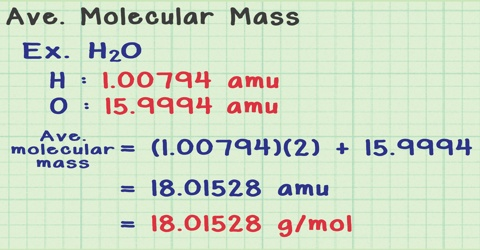Chemistry

# Determination of Molecular Mass from Osmotic Pressure MeasurementsDetermination of Molecular Mass from Osmotic Pressure Measurements

Like the other colligative properties, osmotic pressure measurements are extensively used for the determination of molecular masses of substances. If the number of moles in a solution of volume V liters is ‘n’ then equation (πV = nRT) takes the form,

πV = nRT = w/MRT … … … (1)

where w is the mass of the solute and M its molecular mass. This equation readily gives a method of calculating molecular masses of sours in solution. Equation (1) May also be written as,

r = cRT … … … (2)

where c is the molar concentration.

Example: 2.05 g of an unknown compound is dissolved in 200 mL of solution at 25 °C. If the osmotic pressure is 2.25 atmospheres, calculate the molecular mass of the solute.

Solution:

πV = w/M RT

or, M = wRT / πV

V = 200 mL = 0.2 L

Substituting the values we get,

M = (2.05 x 0.082 x 298) / (2.25 x 0.2) = 111.3 M

The osmotic pressure method is widely used for determination of molecular mass of high polymers like cellulose, proteins, polystyrene etc. The other three colligative properties are not suitable, since the magnitude of lowering of vapour pressure, elevation of boiling point and depression of freezing point is so small that these methods become useless for any practical purpose when the molecular mass of the solute exceeds a few thousand.